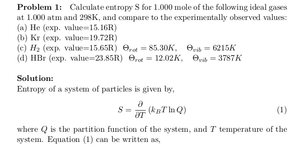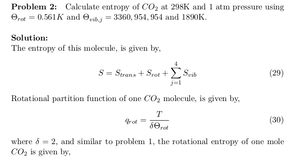Solved Problems in Physics, Physical Chemistry, and Computer Simulation Projects for College and Graduate Students.# Entropy of Monatomic and Diatomic Ideal Gases

Regular price
\$5.99
Sale price
\$5.99
Tax included.

Problem 1:

Calculate entropy S for 1.000 mole of the following ideal gases at 1.000 atm and 298K, and compare to the experimentally observed values:
(a) He (exp. value=15.16R)
(b) Kr (exp. value=19.72R)
(c) H2 (exp. value=15.65R) Θrot = 85.30K, Θvib = 6215K
(d) HBr (exp. value=23.85R) Θrot = 12.02K, Θvib = 3787K

Problem 2:

Calculate entropy of CO2 at 298K and 1 atm pressure using Θrot = 0.561K and Θvib,j = 3360, 954, 954 and 1890K.

By purchasing this product, you will get the step by step solution of the above problems in pdf format and the corresponding latex file where you can edit the solution. I am also available to help  you with any possible question you may have.